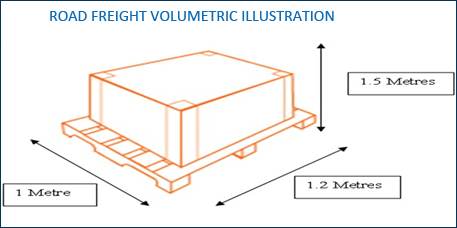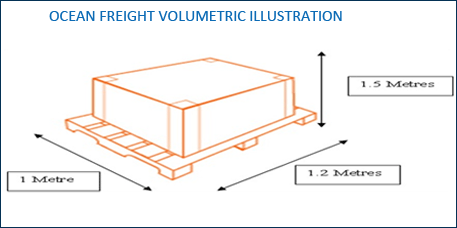﻿ Accutime | Logistics Simplified Distance Calculator

### Logistics Tools

Need help in knowing volumetric weight calculation or Want to know the time in another country or how much a \$ is worth in your local currency? Our Tools are here to help.

### Volumetric Weight Calculation

Dimensional weight is also known as DIM Weight, Volumetric weight, Cubed Weight, etc. Freight carriers utilize the greater of the actual weight or dimensional weight to calculate shipping charges. Dimensional Weight is calculated as (Length x Width x Height) / (Dimensional Factor). Measurements can be made all in inches or all in centimetres, but the appropriate shipping factor must also be used.

#### AIR FREIGHT VOLUMETRIC CALCULATION :

All freight transportation modes are governed by weight and cubic measurement factors, whereby the Carrier will charge on Actual Weight or Volumetric, whichever is deemed to be the greater.
The IATA / Airline Cubic Allowance Factor for Air Freight Shipments is
1 Kilo = 6,000 Cubic Centimetres.

The illustration below shows you how to calculate the Volumetric Chargeable Weight as follows :

• Let’s assume the actual gross weight of this carton is 14 Kilos.
• The carton measures 30 × 45 × 65cms which is 87750 Cubic Centimetres.
• Divide this figure by the IATA/Airline Cubic Allowance Factor of 6000 Cubic Centimetres = 14.625 Kilos.
• In this instance the Air Freight charge will be based on 15 Kilos volumetric, rather than the 14 kilos actual weight.#### CALCULATING CUBIC MEASUREMENT FOR ROAD & OCEAN FREIGHT :

The Standard (RHA-Road Haulage Allowance) Factor for Road Freight is 3 Cubic Metres per 1,000 Kilos, whereas the Standard Maritime Allowance Factor for Ocean LCL Group age Freight is 1 Cubic Metre per 1,000 Kilos. This illustration below shows you how to calculate the Volumetric Chargeable Weight as follows :

OCEAN FREIGHT CALCULATION :
In this instance the Freight Charges for Ocean Freight are calculated as 1.8 Cubic Metres X 1,000 = 1,800 Kgs, so the Chargeable Weight is based on 1,800 Kgs.

CBM CALCULATION FORMULA :
Length (centimetre) x Width (centimetre) x Height (centimetre) / 1,000,000 = Length meter x Width meter x Height meter = Cubic meter (m3) .
e.g. 35 cm x 35 cm x 45 cm = 0.055 cbm

CFT FORMULA :
Length” x Width” x Height” =_________ divided 1728= Cubic feet (CFT)

KT FORMULA :
Pounds divided by 2204.62 = Kiloton (Kt)